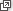## Content

### Factoring

Factoring is the reverse process to expanding. Writing the number 3128 as $$8\times 17 \times 23$$ gives us useful information about the number. For example, it tells us that 3128 is divisible by 8 and 17 and 23. It also allows us to calculate $$3128 \div 23$$ without using a calculator. Similarly, in algebra, factoring an algebraic expression is a very useful and important skill. Indeed, it is very unwise in algebra to expand a complicated collection of brackets unless you have no other option. Factoring is often much the better thing to do. Factoring also enables us to solve certain types of equations and will reappear when we look at quadratic equations and again in the TIMES module Polynomials (Years 9–10).

#### Basic factoring

Students should always be on the lookout for simple common factors.

For example, the terms in the expression $$3x^2+12x$$ have a common factor of $$3x$$ which can be factored out. Thus, $$3x^2+12x=3x(x+4)$$.

Sometimes after doing this we can spot a further common factor. For example,

\begin{align*} 12xy - 15x - 16y + 20 &= 3x(4y - 5) - 4(4y - 5)\\ &= (3x - 4)(4y - 5). \end{align*}
##### Exercise 4

Factorise

$4x^3-12x^2-x+3.$

Hence state the values of $$x$$ which make this expression equal to 0.

A quadratic expression has the form $$ax^2+bx+c$$, where $$a,b,c$$ are given numbers with $$a \ne 0$$. In some cases a quadratic expression can be factored. For example, starting with the expression $$x^2+5x+6$$, we can, rather cleverly, write this as

\begin{align*} x^2+2x+3x+6 &= x(x+2)+3(x+2)\\ &= (x+3)(x+2). \end{align*}

Now how did we know to split the middle term in this way? The answer lies in looking at what happens when we expand:

$(x+a)(x+b) = x^2+(a+b)x+ab.$

Clearly the coefficient of $$x$$ is the sum of $$a$$ and $$b$$ and the constant term is their product. This is why we split the $$5x$$ term into $$2x+3x$$. In practice, when factoring $$x^2+5x+6$$, we seek two integers whose sum is 5 and whose product is 6. Clearly the numbers are 2 and 3, and so we obtain the desired factorisation.

#### Example

Factor

$x^2+23x-50.$

#### Solution

We seek two numbers whose sum is 23 and whose product is $$-50$$. The numbers 25 and $$-2$$ will do. Hence,

$x^2+23x-50 = (x+25)(x-2).$

Provided we can find the appropriate numbers, this method works well for monic quadratics, that is, for quadratics whose leading coefficient is 1.

In the case of a non-monic quadratic, the following algorithm is employed. It is best seen by example.

To factor $$3x^2+14x-5$$, we first multiply the 3 and the $$-5$$ to obtain $$-15$$. Now we find two numbers whose sum is 14 and whose product is $$-15$$. Clearly, these are 15 and $$-1$$. We use these numbers to split the middle term and write

$3x^2+14x-5= 3x^2+15x-x-5.$

Now we factor in pairs, as above. Thus

\begin{align*} 3x^2+14x-5 &= 3x^2+15x-x-5\\ &= 3x(x+5)-1(x+5)\\ &= (3x-1)(x+5). \end{align*}

Of course there are quadratic expressions such as $$x^2+x+1$$ which cannot be factored in this way.

#### Special factorisations

The following three special factorisations are exactly the same as the three special expansions discussed in Expanding (Special expansions) in this module, but used in reverse:

\begin{align*} x^2+2xy+y^2 &= (x+y)^2\\ x^2-2xy+y^2 &= (x-y)^2\\ x^2-y^2 &= (x+y)(x-y). \end{align*}

Of these the last is probably the most important, and students need to spot this factorisation whenever it occurs.

#### Example

Factor

$9x^2-25y^2.$

#### Solution

$$9x^2-25y^2=(3x+5y)(3x-5y)$$.
##### Exercise 5

Factorise fully

$(a^2+ab)^2-(ab+b^2)^2.$

Next page - Content - Algebraic fractions